Related Articles
How to select multiple columns in a pandas dataframe
• Difficulty Level : Easy
• Last Updated : 27 Nov, 2018

Python is a great language for doing data analysis, primarily because of the fantastic ecosystem of data-centric Python packages. Pandas is one of those packages and makes importing and analyzing data much easier.

Let’s discuss all different ways of selecting multiple columns in a pandas DataFrame.

Method #1: Basic Method

Given a dictionary which contains Employee entity as keys and list of those entity as values.

 `# Import pandas package``import` `pandas as pd`` ` `# Define a dictionary containing employee data``data ``=` `{``'Name'``:[``'Jai'``, ``'Princi'``, ``'Gaurav'``, ``'Anuj'``],``        ``'Age'``:[``27``, ``24``, ``22``, ``32``],``        ``'Address'``:[``'Delhi'``, ``'Kanpur'``, ``'Allahabad'``, ``'Kannauj'``],``        ``'Qualification'``:[``'Msc'``, ``'MA'``, ``'MCA'``, ``'Phd'``]}`` ` `# Convert the dictionary into DataFrame ``df ``=` `pd.DataFrame(data)`` ` `# select two columns``df[[``'Name'``, ``'Qualification'``]]`

Output: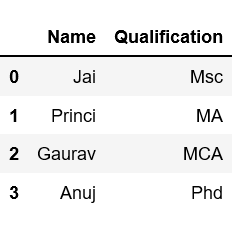Select Second to fourth column.

 `# Import pandas package``import` `pandas as pd`` ` `# Define a dictionary containing employee data``data ``=` `{``'Name'``:[``'Jai'``, ``'Princi'``, ``'Gaurav'``, ``'Anuj'``],``        ``'Age'``:[``27``, ``24``, ``22``, ``32``],``        ``'Address'``:[``'Delhi'``, ``'Kanpur'``, ``'Allahabad'``, ``'Kannauj'``],``        ``'Qualification'``:[``'Msc'``, ``'MA'``, ``'MCA'``, ``'Phd'``]}`` ` `# Convert the dictionary into DataFrame ``df ``=` `pd.DataFrame(data)`` ` `# select all rows ``# and second to fourth column``df[df.columns[``1``:``4``]]`

Output: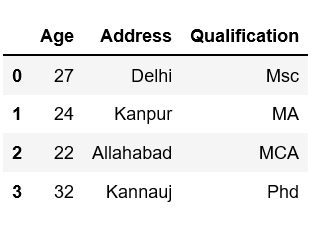Method #2: Using `loc[]`

Example 1: Select two columns

 `# Import pandas package``import` `pandas as pd`` ` `# Define a dictionary containing employee data``data ``=` `{``'Name'``:[``'Jai'``, ``'Princi'``, ``'Gaurav'``, ``'Anuj'``],``        ``'Age'``:[``27``, ``24``, ``22``, ``32``],``        ``'Address'``:[``'Delhi'``, ``'Kanpur'``, ``'Allahabad'``, ``'Kannauj'``],``        ``'Qualification'``:[``'Msc'``, ``'MA'``, ``'MCA'``, ``'Phd'``]}`` ` `# Convert the dictionary into DataFrame ``df ``=` `pd.DataFrame(data)`` ` `# select three rows and two columns``df.loc[``1``:``3``, [``'Name'``, ``'Qualification'``]]`

Output: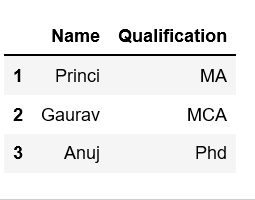Example 2: Select one to another columns. In our case we select column name “Name” to “Address”.

 `# Import pandas package``import` `pandas as pd`` ` `# Define a dictionary containing employee data``data ``=` `{``'Name'``:[``'Jai'``, ``'Princi'``, ``'Gaurav'``, ``'Anuj'``],``        ``'Age'``:[``27``, ``24``, ``22``, ``32``],``        ``'Address'``:[``'Delhi'``, ``'Kanpur'``, ``'Allahabad'``, ``'Kannauj'``],``        ``'Qualification'``:[``'Msc'``, ``'MA'``, ``'MCA'``, ``'Phd'``]}`` ` `# Convert the dictionary into DataFrame ``df ``=` `pd.DataFrame(data)`` ` `# select two rows and ``# column "name" to "Address"``# Means total three columns``df.loc[``0``:``1``, ``'Name'``:``'Address'``]`

Output: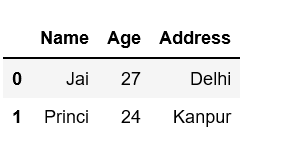Example 3: First filtering rows and selecting columns by label format and then Select all columns.

 `# Import pandas package``import` `pandas as pd`` ` `# Define a dictionary containing employee data``data ``=` `{``'Name'``:[``'Jai'``, ``'Princi'``, ``'Gaurav'``, ``'Anuj'``],``        ``'Age'``:[``27``, ``24``, ``22``, ``32``],``        ``'Address'``:[``'Delhi'``, ``'Kanpur'``, ``'Allahabad'``, ``'Kannauj'``],``        ``'Qualification'``:[``'Msc'``, ``'MA'``, ``'MCA'``, ``'Phd'``]``       ``}``# Convert the dictionary into DataFrame ``df ``=` `pd.DataFrame(data)`` ` `# .loc DataFrame method``# filtering rows and selecting columns by label``# format``# df.loc[rows, columns]``# row 1, all columns``df.loc[``0``, :]`

Output: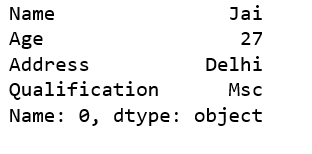Method #3: Using `iloc[]`

Example 1: Select first two column.

 `# Import pandas package``import` `pandas as pd`` ` `# Define a dictionary containing employee data``data ``=` `{``'Name'``:[``'Jai'``, ``'Princi'``, ``'Gaurav'``, ``'Anuj'``],``        ``'Age'``:[``27``, ``24``, ``22``, ``32``],``        ``'Address'``:[``'Delhi'``, ``'Kanpur'``, ``'Allahabad'``, ``'Kannauj'``],``        ``'Qualification'``:[``'Msc'``, ``'MA'``, ``'MCA'``, ``'Phd'``]}`` ` `# Convert the dictionary into DataFrame ``df ``=` `pd.DataFrame(data)`` ` `# Remember that Python does not``# slice inclusive of the ending index.``# select all rows ``# select first two column``df.iloc[:, ``0``:``2``] `

Output: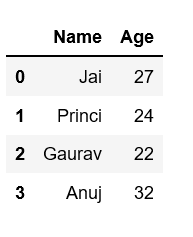Example 2: Select all or some columns, one to another using .iloc.

 `# Import pandas package``import` `pandas as pd`` ` `# Define a dictionary containing employee data``data ``=` `{``'Name'``:[``'Jai'``, ``'Princi'``, ``'Gaurav'``, ``'Anuj'``],``        ``'Age'``:[``27``, ``24``, ``22``, ``32``],``        ``'Address'``:[``'Delhi'``, ``'Kanpur'``, ``'Allahabad'``, ``'Kannauj'``],``        ``'Qualification'``:[``'Msc'``, ``'MA'``, ``'MCA'``, ``'Phd'``]}`` ` `# Convert the dictionary into DataFrame ``df ``=` `pd.DataFrame(data)`` ` `# iloc[row slicing, column slicing]``df.iloc [``0``:``2``, ``1``:``3``]`

Output: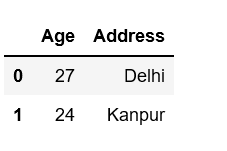Method #4: Using `.ix`

Select all or some columns, one to another using `.ix`.

 `# Import pandas package``import` `pandas as pd`` ` `# Define a dictionary containing employee data``data ``=` `{``'Name'``:[``'Jai'``, ``'Princi'``, ``'Gaurav'``, ``'Anuj'``],``        ``'Age'``:[``27``, ``24``, ``22``, ``32``],``        ``'Address'``:[``'Delhi'``, ``'Kanpur'``, ``'Allahabad'``, ``'Kannauj'``],``        ``'Qualification'``:[``'Msc'``, ``'MA'``, ``'MCA'``, ``'Phd'``]}`` ` `# Convert the dictionary into DataFrame ``df ``=` `pd.DataFrame(data)`` ` `# select all rows and 0 to 2 columns ``print``(df.ix[:, ``0``:``2``])`

Output: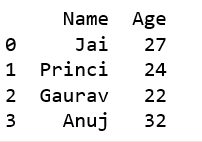Attention geek! Strengthen your foundations with the Python Programming Foundation Course and learn the basics.

To begin with, your interview preparations Enhance your Data Structures concepts with the Python DS Course. And to begin with your Machine Learning Journey, join the Machine Learning – Basic Level Course

My Personal Notes arrow_drop_up